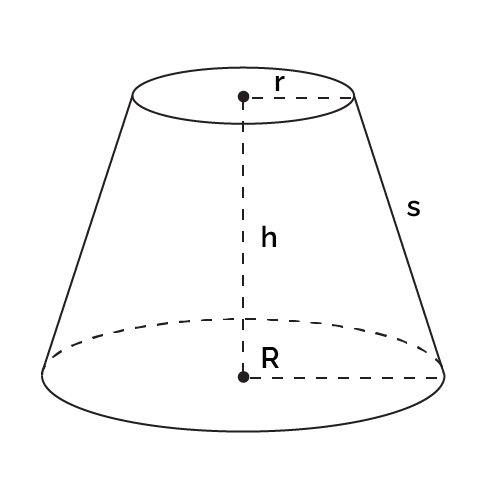# Truncated Cone Volume Calculator

Created by Anna Szczepanek, PhD
Reviewed by Davide Borchia
Last updated: Aug 22, 2022

With this Omni's truncated cone volume calculator, you will never again have to wonder how to calculate the volume of a truncated cone. Here you'll find the instruction on how to apply the truncated cone volume formula as well as a step-by-step example.

## Truncated cone volume formula

Recall a truncated cone (a.k.a. frustum) is a cone whose top is cut off with a plane parallel to the base. This definition gives us immediately a method of calculating the truncated cone volume: we need to subtract the volume of the smaller cone (the one we cut off) from the volume of the bigger (original) cone. What is left is the volume of the truncated cone. Easy!

Following this line of reasoning and performing some basic math operations, we arrive at the following volume formula for our truncated cone:

V = (1/3) * π * h * (r² + r * R + R²),

Where:

• R is the radius of the base of the original cone (bottom surface);
• r is the radius of the top surface; and
• h is the height of our truncated cone.## How to use this truncated cone volume calculator?

To compute the volume of a truncated cone with our tool, you need to:

1. Insert the data you have. Most probably, you know the height h and the top and bottom radii: r and R, respectively.
2. It may happen, in more advanced problems, that you know the slant height s. Insert whatever you have!
3. The Omni magic happens, and the empty fields, including volume, fill in immediately.
4. Adjust the units if needed.

As you can see, Omni's truncated cone volume calculator is a very straightforward tool!

## Similar Omni tools

Want to learn more about solids and truncated cones in particular? Make sure to check out the following Omni tools:

## FAQ

### What is the volume of the frustum with height 5 cm and radii 1 cm and 2 cm?

36.65 cm³. To get this result you need to remember the truncated cone volume formula: V = (1/3) * π * h * (r² + r * R + R²). Plugging in h = 5, r = 1, and R = 2, we arrive at the desired result.

### How do I calculate the volume of a truncated cone?

To calculate the volume of a truncated cone, follow these steps:

1. Determine the top and bottom radii of your frustum: r and R, respectively.
2. We will also need the height h of the solid (the perpendicular distance between the two parallel surfaces).
3. Now, apply the formula: V = (1/3) * π * h * (r² + r * R + R²), where π ≈ 3.14.
4. That's it! You've just determined the volume of your frustum.
Anna Szczepanek, PhD
Truncated cone data
in
in
Height (h)
in
Slant height (s)
inVolume
cu in
People also viewed…

### Inverse matrix

The inverse matrix calculator will check if the square matrix you give it has an inverse, and, if it does, will calculate it in a few easy steps.

### Meat footprint

Check out the meat impact - on the environment and your health.

### Radius of a sphere

How to find the radius of a sphere? What's the radius of a sphere formula? Check out this radius of a sphere calculator and answer these questions.

### Snowman

The perfect snowman calculator uses math & science rules to help you design the snowman of your dreams!